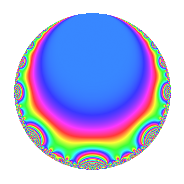# Properties

 Label 8008.2.a.hLevel 8008 Weight 2 Character orbit 8008.a Self dual Yes Analytic conductor 63.944 Analytic rank 0 Dimension 2 CM No Inner twists 1

# Related objects

## Newspace parameters

 Level: $$N$$ = $$8008 = 2^{3} \cdot 7 \cdot 11 \cdot 13$$ Weight: $$k$$ = $$2$$ Character orbit: $$[\chi]$$ = 8008.a (trivial)

## Newform invariants

 Self dual: Yes Analytic conductor: $$63.9442019386$$ Analytic rank: $$0$$ Dimension: $$2$$ Coefficient field: $$\Q(\sqrt{13})$$ Coefficient ring: $$\Z[a_1, a_2, a_3]$$ Coefficient ring index: $$1$$ Fricke sign: $$-1$$ Sato-Tate group: $\mathrm{SU}(2)$

## $q$-expansion

Coefficients of the $$q$$-expansion are expressed in terms of $$\beta = \frac{1}{2}(1 + \sqrt{13})$$. We also show the integral $$q$$-expansion of the trace form.

 $$f(q)$$ $$=$$ $$q + ( 1 + \beta ) q^{3} + ( 2 + \beta ) q^{5} + q^{7} + ( 1 + 3 \beta ) q^{9} +O(q^{10})$$ $$q + ( 1 + \beta ) q^{3} + ( 2 + \beta ) q^{5} + q^{7} + ( 1 + 3 \beta ) q^{9} - q^{11} - q^{13} + ( 5 + 4 \beta ) q^{15} + ( -3 + \beta ) q^{17} + ( 2 - 3 \beta ) q^{19} + ( 1 + \beta ) q^{21} -8 q^{23} + ( 2 + 5 \beta ) q^{25} + ( 7 + 4 \beta ) q^{27} + 4 q^{29} + ( -4 + 4 \beta ) q^{31} + ( -1 - \beta ) q^{33} + ( 2 + \beta ) q^{35} + ( 4 + 2 \beta ) q^{37} + ( -1 - \beta ) q^{39} -2 \beta q^{41} + ( 1 - 3 \beta ) q^{43} + ( 11 + 10 \beta ) q^{45} + ( 4 - 4 \beta ) q^{47} + q^{49} -\beta q^{51} + ( 5 + 3 \beta ) q^{53} + ( -2 - \beta ) q^{55} + ( -7 - 4 \beta ) q^{57} + ( -6 + 4 \beta ) q^{59} + ( 3 - 7 \beta ) q^{61} + ( 1 + 3 \beta ) q^{63} + ( -2 - \beta ) q^{65} + ( 7 + \beta ) q^{67} + ( -8 - 8 \beta ) q^{69} -7 \beta q^{71} + ( -6 + 2 \beta ) q^{73} + ( 17 + 12 \beta ) q^{75} - q^{77} + ( -2 - 3 \beta ) q^{79} + ( 16 + 6 \beta ) q^{81} + ( 14 - 3 \beta ) q^{83} -3 q^{85} + ( 4 + 4 \beta ) q^{87} + ( 3 + \beta ) q^{89} - q^{91} + ( 8 + 4 \beta ) q^{93} + ( -5 - 7 \beta ) q^{95} -10 q^{97} + ( -1 - 3 \beta ) q^{99} +O(q^{100})$$ $$\operatorname{Tr}(f)(q)$$ $$=$$ $$2q + 3q^{3} + 5q^{5} + 2q^{7} + 5q^{9} + O(q^{10})$$ $$2q + 3q^{3} + 5q^{5} + 2q^{7} + 5q^{9} - 2q^{11} - 2q^{13} + 14q^{15} - 5q^{17} + q^{19} + 3q^{21} - 16q^{23} + 9q^{25} + 18q^{27} + 8q^{29} - 4q^{31} - 3q^{33} + 5q^{35} + 10q^{37} - 3q^{39} - 2q^{41} - q^{43} + 32q^{45} + 4q^{47} + 2q^{49} - q^{51} + 13q^{53} - 5q^{55} - 18q^{57} - 8q^{59} - q^{61} + 5q^{63} - 5q^{65} + 15q^{67} - 24q^{69} - 7q^{71} - 10q^{73} + 46q^{75} - 2q^{77} - 7q^{79} + 38q^{81} + 25q^{83} - 6q^{85} + 12q^{87} + 7q^{89} - 2q^{91} + 20q^{93} - 17q^{95} - 20q^{97} - 5q^{99} + O(q^{100})$$

## Embeddings

For each embedding $$\iota_m$$ of the coefficient field, the values $$\iota_m(a_n)$$ are shown below.

For more information on an embedded modular form you can click on its label.

Label $$\iota_m(\nu)$$ $$a_{2}$$ $$a_{3}$$ $$a_{4}$$ $$a_{5}$$ $$a_{6}$$ $$a_{7}$$ $$a_{8}$$ $$a_{9}$$ $$a_{10}$$
1.1
 −1.30278 2.30278
0 −0.302776 0 0.697224 0 1.00000 0 −2.90833 0
1.2 0 3.30278 0 4.30278 0 1.00000 0 7.90833 0
 $$n$$: e.g. 2-40 or 990-1000 Significant digits: Format: Complex embeddings Normalized embeddings Satake parameters Satake angles

## Inner twists

This newform does not admit any (nontrivial) inner twists.

## Atkin-Lehner signs

$$p$$ Sign
$$2$$ $$1$$
$$7$$ $$-1$$
$$11$$ $$1$$
$$13$$ $$1$$

## Hecke kernels

This newform can be constructed as the intersection of the kernels of the following linear operators acting on $$S_{2}^{\mathrm{new}}(\Gamma_0(8008))$$:

 $$T_{3}^{2} - 3 T_{3} - 1$$ $$T_{5}^{2} - 5 T_{5} + 3$$ $$T_{17}^{2} + 5 T_{17} + 3$$